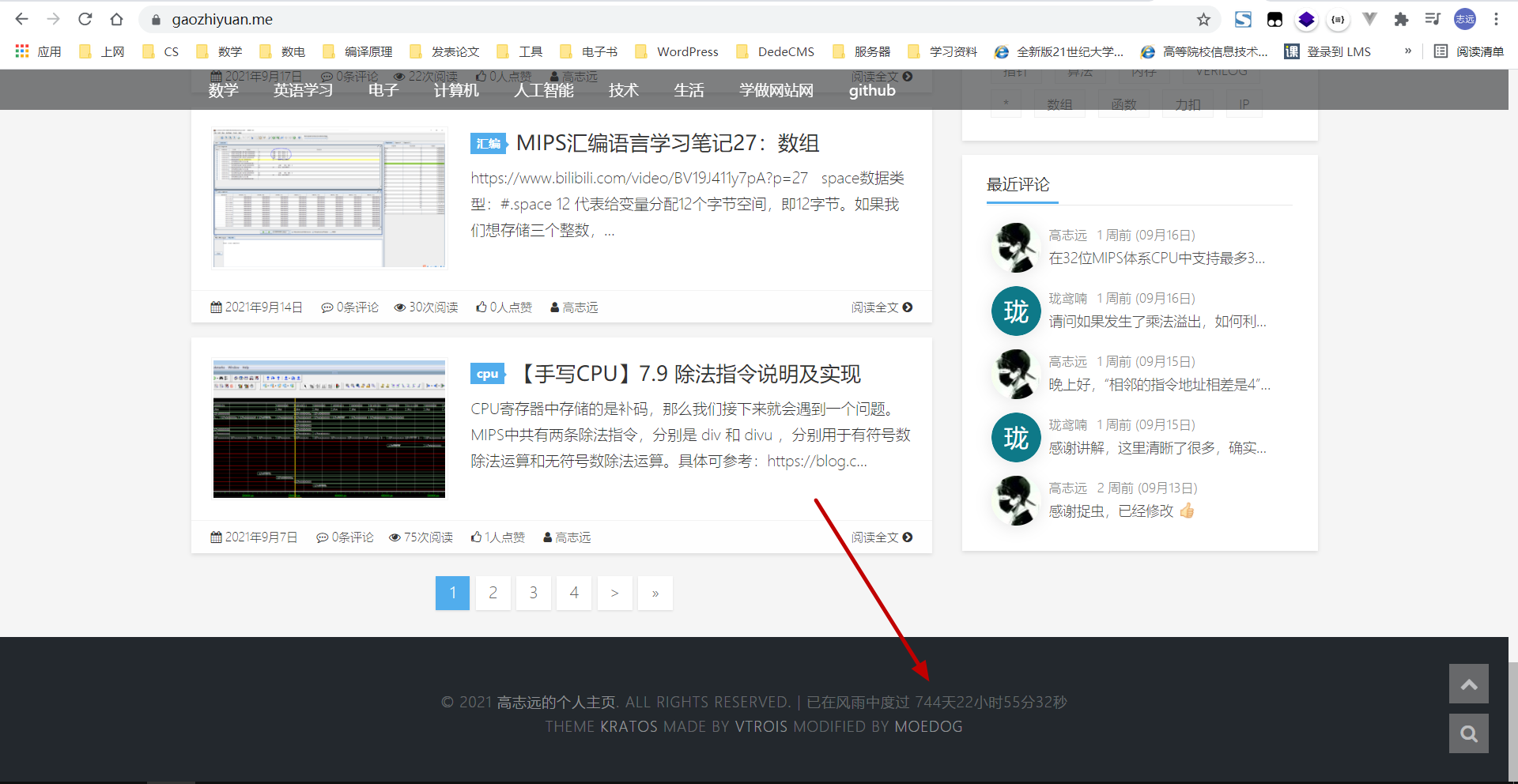# 如何让网站自动统计运行天数，显示本站已运行XX天，网站运行天数统计代码

1. 首页
2. »
3. 图文教程
4. »
5. WordPress建站教程
6. »
7. 如何让网站自动统计运行天数，显示本站已运行XX天，网站运行天数统计代码

## 文章目录## 实现代码

<?php
// 设置时区
date_default_timezone_set(‘Asia/Shanghai’);
function Sec2Time(\$time){
if(is_numeric(\$time)){
\$value = array(
“years” => 0, “days” => 0, “hours” => 0,
“minutes” => 0, “seconds” => 0,
);
if(\$time >= 31556926){
\$value[“years”] = floor(\$time/31556926);
\$time = (\$time%31556926);
}
if(\$time >= 86400){
\$value[“days”] = floor(\$time/86400);
\$time = (\$time%86400);
}
if(\$time >= 3600){
\$value[“hours”] = floor(\$time/3600);
\$time = (\$time%3600);
}
if(\$time >= 60){
\$value[“minutes”] = floor(\$time/60);
\$time = (\$time%60);
}
\$value[“seconds”] = floor(\$time);
return (array) \$value;
}else{
return (bool) FALSE;
}
}
// 本站创建的时间
\$site_create_time = strtotime(‘2017-09-01 00:00:00’);
\$time = time() – \$site_create_time;
\$uptime = Sec2Time(\$time);
?>

### Astra WooCommerce 产品分类页侧边栏Categories如何显示子分类